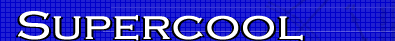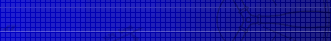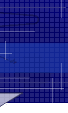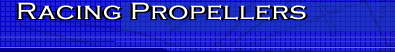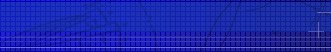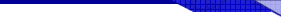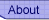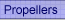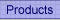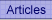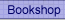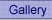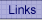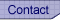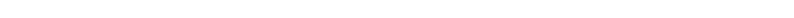﻿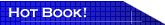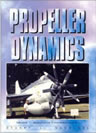Propeller Dynamics Essential reading for model aircraft contest fliers. This is the only book on the market explaining propeller theory in non-mathematical terms. A rattling good read, I know, I wrote it. Read more...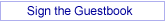## Power absorption and thrust.

Subsequent to last months tips on transonic airfoil sections suitable for F2A and F3D propellers, Charlie Stone was kind enough to fax me details of Luis Paramon's 1996 World champion F2A model and its propeller. Regrettably the translation is awful, so that details of the airfoil sections remain largely hidden. However, it appears that the low Mach sections are of the Clark Y type (t/c = 11.72%), which is your typical flat bottom section, while the tips are thinned to 7%. The tip airfoil appears to progress from the Clark Y to a supersonic diamond section with the high-point at 50%: the leading and trailing edges are sharp.

This is interesting, as the tip Mach number is 0.87 at the 74.5mm radius. Diamond sections require fully supersonic flow to work properly, so the use at M = .87 is novel. My guess is that a 6% ARA-D section would be better, but the diamond shape is easy to carve into the prop blank, while the ARA section is difficult to reproduce. There is really no resemblance between ARA-D and the diamond section, so it may be worth my while machining up Luis' prop with the ARA-D section and giving that a whirl.

The radial distribution of chord is nearly parallel for most of the prop, being 13.5mm at the 50% station, 12.8 at 75% and 8.4 at the tip. This at 38000 RPM and 300 KPH gives Reynolds numbers of 170000, 208000 and 173000 respectively; these are good, the bad stuff starts at 150000 and less. The tip is squared off in the computer display and the photograph, but is claimed to be of the scimitar shape in the text. All very interesting.

Now this month I promised you something on power absorption and thrust. There are 2 really neat formulae for propellers which have been known since at least 1889 and may be attributable to the Frenchman Renard. With P for power absorption, T for thrust, n for RPM and D for diameter, they are:

P = Cp * n^3 * D^5

and

T = Ct * n^2 * D^4

The units don't matter much, as they are swallowed up in the coefficients Cp and Ct. If you don't know what a coefficient is, don't lose any sleep over it. It's just a constant number, chosen to make sure the equation gives the right answer! Even in the above equations, the coefficients are pretty flakey: if you look at all closely at the theory, you find that they are slowly varying functions of Mach and Reynolds numbers, so they are not even constants at all.
Despite this, they retain some interest.

Suppose you "hot" up your engine and gain 500 RPM. Well, this must
be a power gain, but how much? We'll cheat a little and say that the above equations work for static measurements on your bench, which won't be too far wrong.

Before the rework your RPM was 22000, and after it was 22500.
Then we can write:

Pi = Cp * 22000^3 * D^5

where Pi means initial power before rework.

Pf = Cp * 22500^3 * D^5

where Pf means power after rework.
Then

Pf / Pi = (Cp * 22500^3 * D^5) / (Cp * 22000^3 * D^5)

Things the same top and bottom cancel out leaving

Pf /Pi = 22500^3 / 22000^3
= 1.07

which is a 7% gain in power. Using the second equation, we can
write

Tf / Ti = (Ct * 22500^2 * D^4) / (Ct * 22000^2 * D^4)
= 1.046

which is a 4.6% gain in thrust. Since speed varies with the square root of the thrust, this could mean an increase in speed of 2.27%.

I'll leave you to try the same calculation, but varying the diameter instead. You'll find that extra diameter requires heaps of extra power to get the same RPM.
Time to sign off, I hope the editor can fit in the ARA-D sections left over from last month.